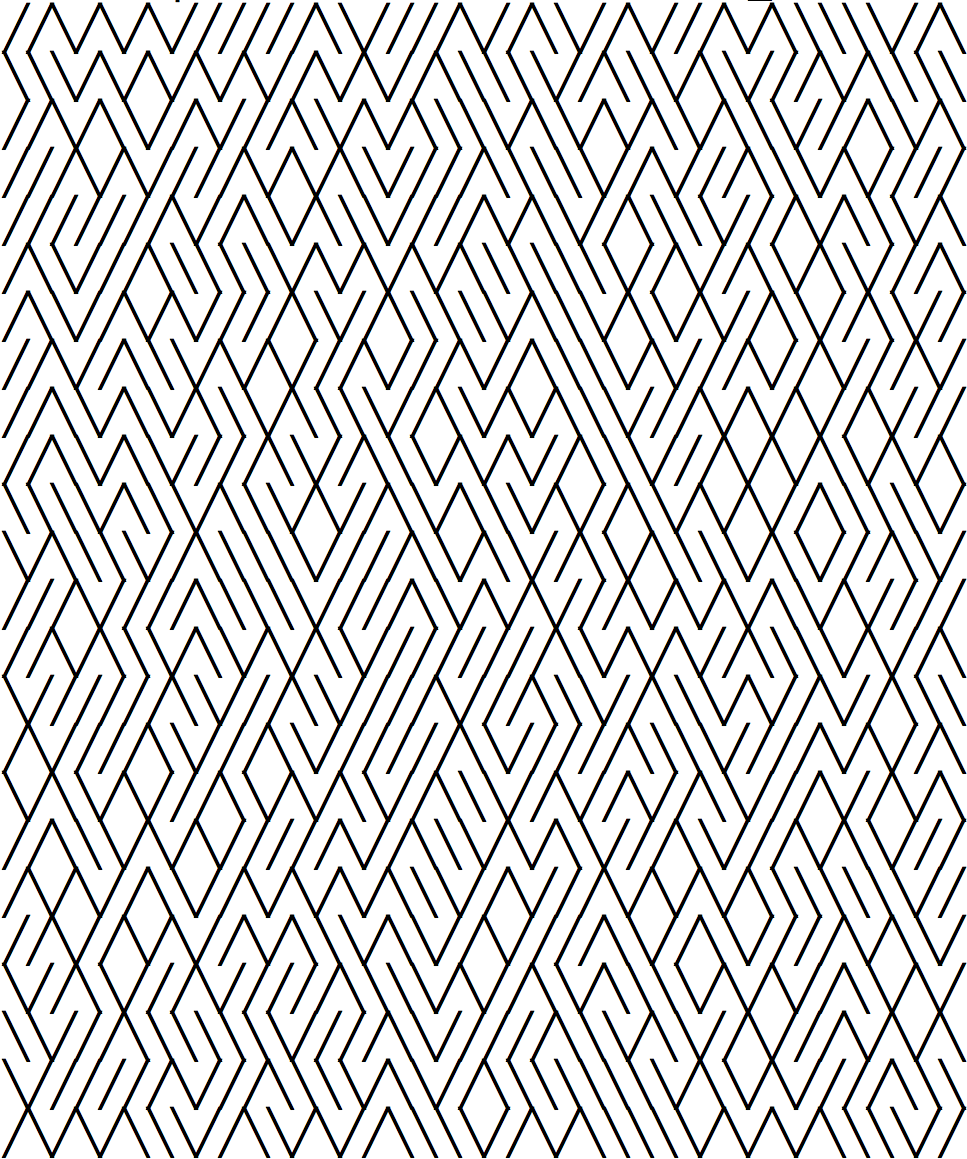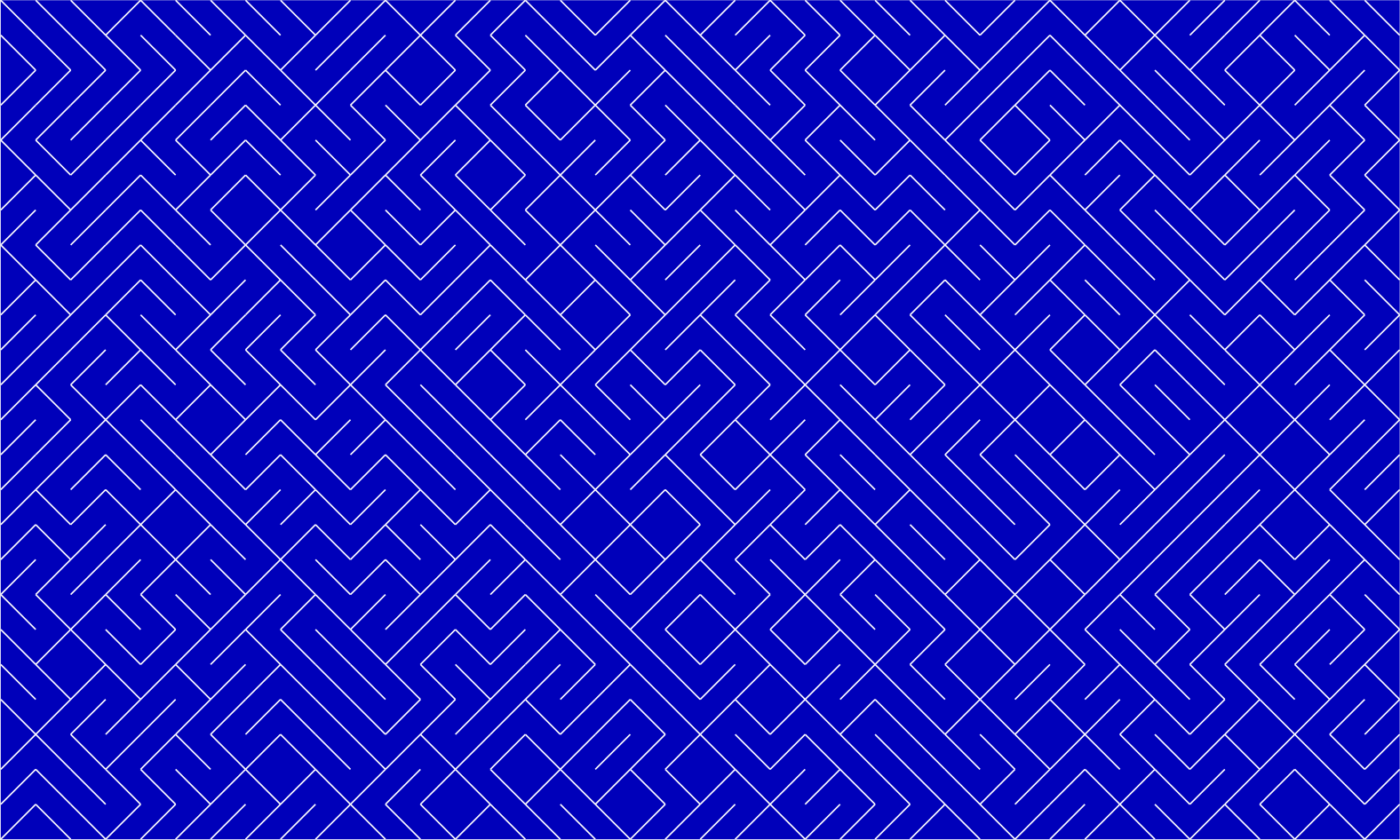# “10 PRINT” in TikZ

The book 10 PRINT CHR$(205.5+RND(1)); : GOTO 10, by Nick Montfort, et. al., uses a one-line Commodore 64 BASIC program “as a gateway into a deeper understanding of how computing works in society and what the writing, reading, and execution of computer code mean” (p. 4). The focus is on the titular program, which the authors call 10 PRINT for short: 10 PRINT CHR$(205.5+RND(1)); : GOTO 10

This program prints an infinite random sequence made up of the box-drawing characters ╱ and ╲ (that’s U+2571 and U+2572, respectively, encoded in the Commodore character set PETSCII as 205 and 206.), in order to make a random maze. This produces an output similar to the following, except that the characters on a Commodore screen are more square:(The book’s web site gives a better idea how the output on a Commodore would look.)

It’s not hard to reproduce 10 PRINT in Python, albeit using more than one line:

 1 2 3 4 5 6 7  #! /usr/bin/env python3 import random for j in range(0, 24): print("".join([random.choice(["\u2571","\u2572"]) for k in range(0, 40)]))

(Instead of an infinite string, my program only outputs a 40 × 24 block of characters.) To get the output to look right, you might need to mess with the font settings in your Terminal. I found that that Melno Regular font on my Mac works.

But I really thought it would be more fun to obtain a 10 PRINT-like output using TikZ, as TikZ is capable of generating random numbers! Instead of using the characters ╱ and ╲, I will draw the lines directly:

  1 2 3 4 5 6 7 8 9 10 11 12 13 14 15 16 17 18 19 20 21 22 23 24 25 26 27 28 29  \documentclass{standalone} \usepackage{tikz} \begin{document} \begin{tikzpicture} % Draw background \fill[blue!75!black] (0, 0) -- (0, 24) -- (40, 24) -- (40, 0) -- cycle; % Draw maze \foreach \y in {0, ..., 23}{ \foreach \x in {0, ..., 39}{ % Randomly choose a = 0 or a = 1 \pgfmathrandominteger{\a}{0}{1}; % If a = 0, then will draw SW-NE line % If a = 1, then will draw NW-SE line \draw[very thick, white] (\x, \y + \a) -- (\x + 1, \y + 1 - \a); } } \end{tikzpicture} \end{document}

I really like the output: